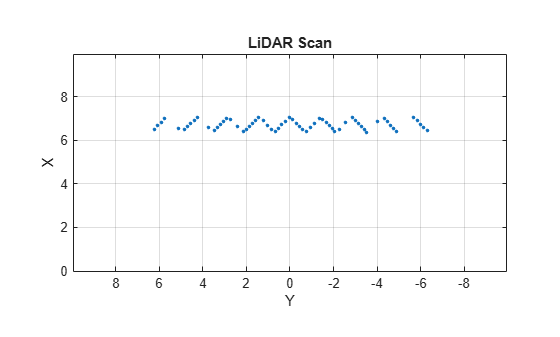# rangeSensor

## Description

The `rangeSensor` System object™ is a range-bearing sensor that is capable of outputting range and angle measurements based on the given sensor pose and occupancy map. The range-bearing readings are based on the obstacles in the occupancy map.

To simulate a range-bearing sensor using this object:

1. Create the `rangeSensor` object and set its properties.

2. Call the object with arguments, as if it were a function.

## Creation

### Syntax

``rbsensor = rangeSensor``
``rbsensor = rangeSensor(Name,Value)``

### Description

example

````rbsensor = rangeSensor` returns a `rangeSensor` System object, `rbsensor`. The sensor is capable of outputting range and angle measurements based on the sensor pose and an occupancy map.```
````rbsensor = rangeSensor(Name,Value)` sets properties for the sensor using one or more name-value pairs. Unspecified properties have default values. Enclose each property name in quotes.```

## Properties

expand all

Unless otherwise indicated, properties are nontunable, which means you cannot change their values after calling the object. Objects lock when you call them, and the `release` function unlocks them.

If a property is tunable, you can change its value at any time.

The minimum and maximum detectable range, specified as a 1-by-2 positive real-valued vector. Units are in meters.

Example: `[1 15]`

Tunable: Yes

Data Types: `single` | `double`

Minimum and maximum horizontal detection angle, specified as a 1-by-2 real-valued vector. Units are in radians.

Example: `[-pi/3 pi/3]`

Data Types: `single` | `double`

Resolution of horizontal angle readings, specified as a positive scalar. The resolution defines the angular interval between two consecutive sensor readings. Units are in radians.

Example: `0.01`

Data Types: `single` | `double`

The standard deviation of range noise, specified as a positive scalar. The range noise is modeled as a zero-mean white noise process with the specified standard deviation. Units are in meters.

Example: `0.01`

Tunable: Yes

Data Types: `single` | `double`

The standard deviation of horizontal angle noise, specified as a positive scalar. The range noise is modeled as a zero-mean white noise process with the specified standard deviation. Units are in radians.

Example: `0.01`

Tunable: Yes

Data Types: `single` | `double`

Number of output readings for each pose of the sensor, specified as a positive integer. This property depends on the `HorizonalAngle` and `HorizontalAngleResolution` properties.

Data Types: `single` | `double`

## Usage

### Syntax

``[ranges,angles] = rbsensor(pose,map)``

### Description

````[ranges,angles] = rbsensor(pose,map)` returns the range and angle readings from the 2-D pose information and the ground-truth map. ```

### Input Arguments

expand all

Poses of the sensor in the 2-D map, specified as an N-by-3 real-valued matrix, where N is the number of poses to simulate the sensor. Each row of the matrix corresponds to a pose of the sensor in the order of [x, y, θ]. x and y represent the position of the sensor in the map frame. The units of x and y are in meters. θ is the heading angle of the sensor with respect to the positive x-direction of the map frame. The units of θ are in radians.

Ground-truth map, specified as an `occupancyMap` (Navigation Toolbox) or a `binaryOccupancyMap` (Navigation Toolbox) object. For the `occupancyMap` input, the range-bearing sensor considers a cell as occupied and returns a range reading if the occupancy probability of the cell is greater than the value specified by the `OccupiedThreshold (Navigation Toolbox)` property of the occupancy map.

### Output Arguments

expand all

Range readings, specified as an R-by-N real-valued matrix. N is the number of poses for which the sensor is simulated, and R is the number of sensor readings per pose of the sensor. R is same as the value of the `NumReadings` property.

Angle readings, specified as an R-by-1 real-valued vector. R is the number of sensor readings per pose of the sensor. R is same as the value of the `NumReadings` property.

## Object Functions

To use an object function, specify the System object as the first input argument. For example, to release system resources of a System object named `obj`, use this syntax:

`release(obj)`

expand all

 `step` Run System object algorithm `clone` Create duplicate System object

## Examples

collapse all

Create a range-bearing sensor.

`rbsensor = rangeSensor;`

Specify the pose of the sensor and the ground-truth map.

```truePose = [0 0 pi/4]; trueMap = binaryOccupancyMap(eye(10));```

`[ranges, angles] = rbsensor(truePose, trueMap);`

Visualize the results using `lidarScan`.

```scan = lidarScan(ranges, angles); figure plot(scan)```## Extended Capabilities